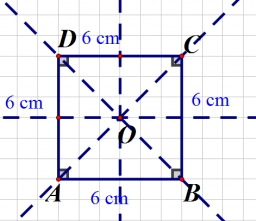# Calculate 8166

Calculate the perimeter of a square when we know the length of its diagonal e = 4.2 m

o =  11.8794 m

### Step-by-step explanation:Did you find an error or inaccuracy? Feel free to write us. Thank you!

Tips to related online calculators
Pythagorean theorem is the base for the right triangle calculator.